Question

Describe the preparation of 3.00 L of 0.150 M glycine buffer, pH 8.6, from glycine and 1.00 M NaOH. What mass of glycine is required? The appropriate pKa of glycine is 9.6. Express your answer using three significant figures.

We need at least 10 more requests to produce the answer.

0 / 10 have requested this problem solution

The more requests, the faster the answer.

All students who have requested the answer will be notified once they are available.

#### Earn Coins

Coins can be redeemed for fabulous gifts.

Similar Homework Help Questions
• ### 1) Using a 0.25 M phosphate buffer with a pH of 7.2, you add 0.79 mL of 0.48 M HCl to 54 mL of the buffer. What is the n...

1) Using a 0.25 M phosphate buffer with a pH of 7.2, you add 0.79 mL of 0.48 M HCl to 54 mL of the buffer. What is the new pH of the solution? (Enter your answer to three significant figures.) 2) Using a 0.25 M phosphate buffer with a pH of 7.2, you add 0.79 mL of 0.48 M NaOH to 54 mL of the buffer. What is the new pH of the solution? (Enter your answer to three...

• ### Part A Calculate the pH of 0.250 L of a 0.36 M formic acid-0.30 M sodium...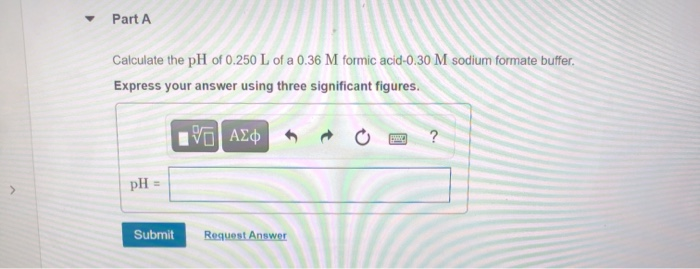Part A Calculate the pH of 0.250 L of a 0.36 M formic acid-0.30 M sodium formate buffer. Express your answer using three significant figures. ΑΣΦ pH = > Submit Request Answer Part B After the addition of 0.0050 mol of NaOH. Assume that the volume remains constant Express your answer using three significant figures. VALO o ? pH = Submit Request Answer Part C After the addition of 0.0050 mol of HCl. Assume that the volume remains constant. Express...

• ### (3 pts) 4. You are asked to prepare a 0.100 M)glycine buffer pH 9.0. You are given a bottle of glycine (FW 75.1 g/mol) and a stock solution of 1.00 M NaOH. How many grams of glycine and how many...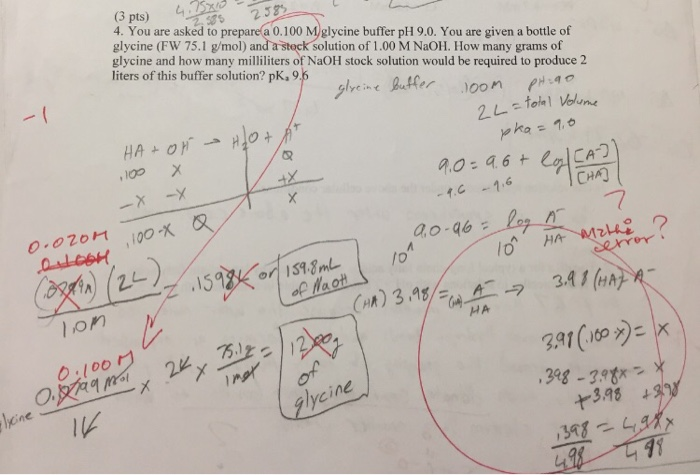(3 pts) 4. You are asked to prepare a 0.100 M)glycine buffer pH 9.0. You are given a bottle of glycine (FW 75.1 g/mol) and a stock solution of 1.00 M NaOH. How many grams of glycine and how many milliliters of NaOH stock solution would be required to produce 2 liters of this buffer solution? pk,95 alyeine tr oon 2 38 CHA) 7 3A1 (HA o.l0o glycine 3.98 (3 pts) 4. You are asked to prepare a 0.100 M)glycine...

• ### Calculate the pH of 1.00 L of a buffer that is 1.00 M in acetic acid...

Calculate the pH of 1.00 L of a buffer that is 1.00 M in acetic acid and 1.00 M in sodium acetate after the addition of 0.150 mole of NaOH.

• ### Solution A is a 1.00 L buffer solution that is 1.196 M in acetic acid and...

Solution A is a 1.00 L buffer solution that is 1.196 M in acetic acid and 1.196 M in sodium acetate. Acetic acid has a pKa of 4.74. What is the pH change of this solution upon addition of 0.1 mol of HCl? Enter your answer numerically to three significant figures.

• ### You are asked to prepare 500. mL 0.150 M acetate buffer at pH 5.10 using only...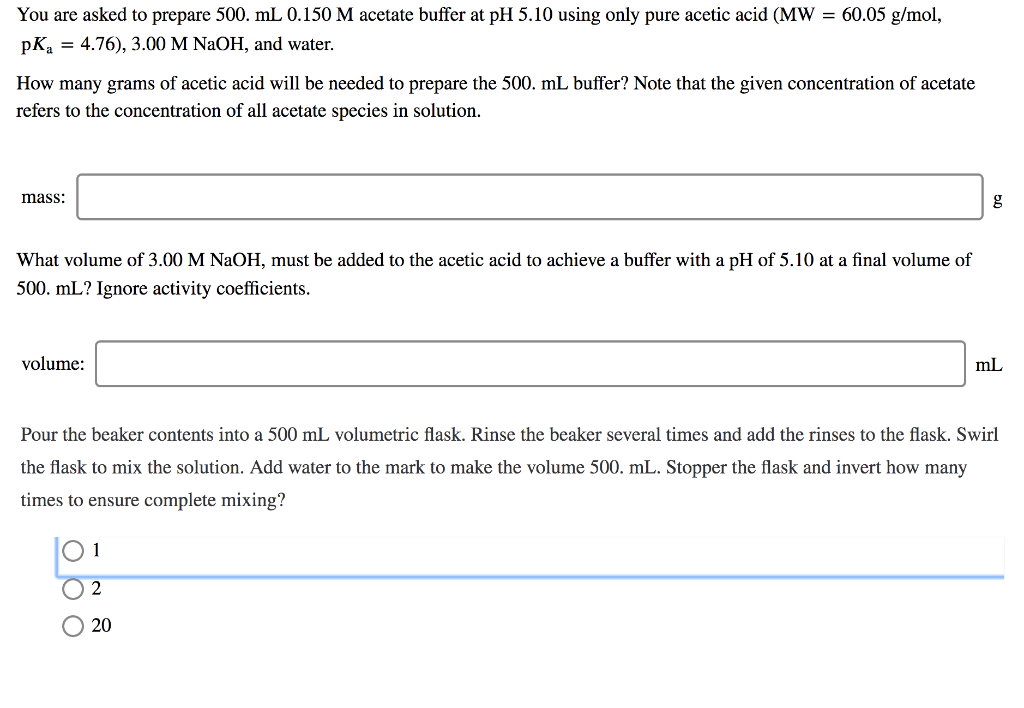You are asked to prepare 500. mL 0.150 M acetate buffer at pH 5.10 using only pure acetic acid (MW = 60.05 g/mol, pka = 4.76), 3.00 M NaOH, and water. How many grams of acetic acid will be needed to prepare the 500. mL buffer? Note that the given concentration of acetate refers to the concentration of all acetate species in solution. mass: DD What volume of 3.00 M NaOH, must be added to the acetic acid to achieve...

• ### You are asked to prepare 500. mL 0.150 M acetate buffer at pH 5.00 using only...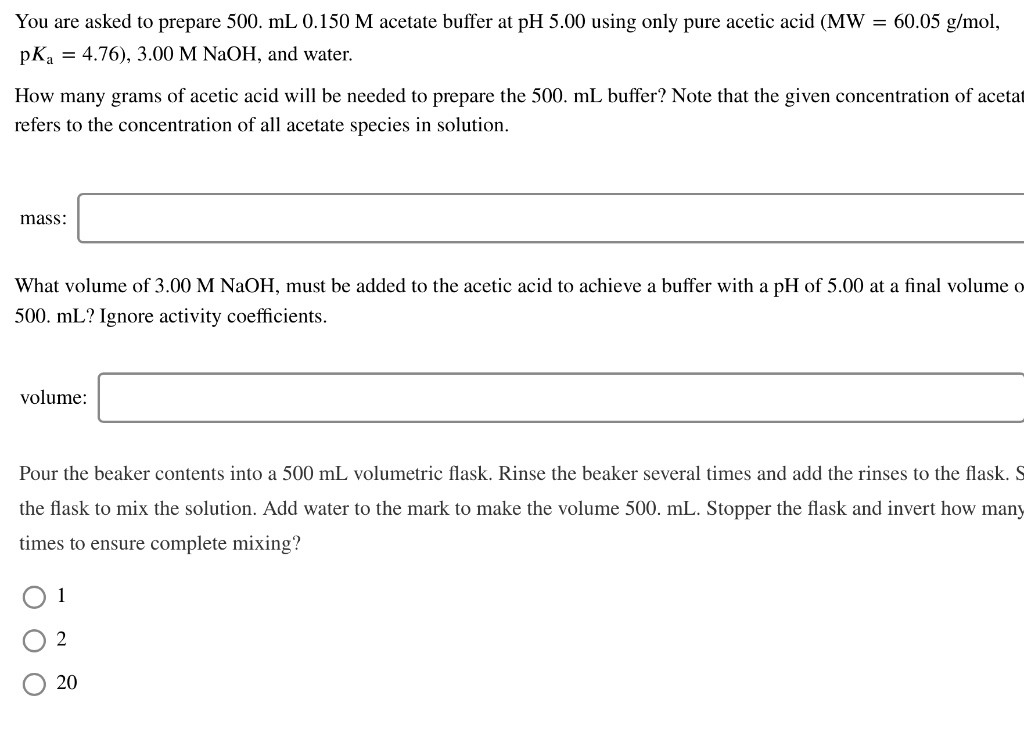You are asked to prepare 500. mL 0.150 M acetate buffer at pH 5.00 using only pure acetic acid (MW = 60.05 g/mol, pKa = 4.76), 3.00 M NaOH, and water. How many grams of acetic acid will be needed to prepare the 500. mL buffer? Note that the given concentration of acetat refers to the concentration of all acetate species in solution. mass: What volume of 3.00 M NaOH, must be added to the acetic acid to achieve a...

• ### 1. Calculate the pH of a buffer solution prepared by mixing 0.250 L of 0.150 M...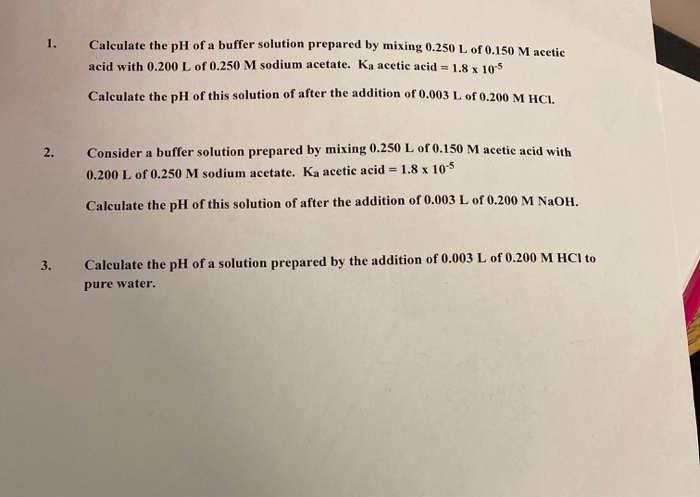1. Calculate the pH of a buffer solution prepared by mixing 0.250 L of 0.150 M acetic acid with 0.200 L of 0.250 M sodium acetate. Ka acetic acid = 1.8 x 10-5 Calculate the pH of this solution of after the addition of 0.003 L of 0.200 M HCL 2. Consider a buffer solution prepared by mixing 0.250 L of 0.150 M acetic acid with 0.200 L of 0.250 M sodium acetate. Ka acetic acid = 1.8 x 10-5...

• ### Using a 0.25 M phosphate buffer with a pH of 7.2, you add 0.75 mL of 0.55 M HCl to 57 mL of the buffer. What is the new...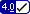Using a 0.25 M phosphate buffer with a pH of 7.2, you add 0.75 mL of 0.55 M HCl to 57 mL of the buffer. What is the new pH of the solution? (Enter your answer to three significant figures.) Using a 0.25 M phosphate buffer with a pH of 7.2, you add 0.75 mL of 0.55 M NaOH to 57 mL of the buffer. What is the new pH of the solution? (Enter your answer to three significant figures.)

• ### C. A 1.00 L buffer solution is 0.150 M in HC7H5O2 and 0.250 M in LiC7H502....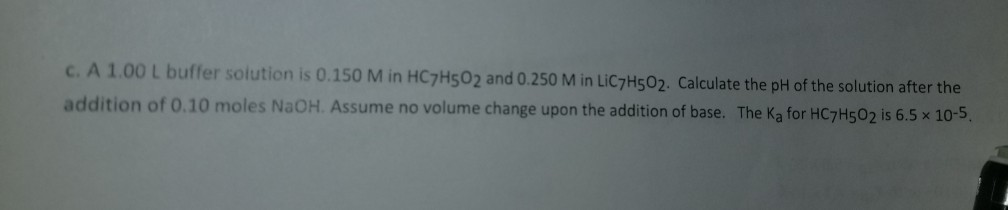C. A 1.00 L buffer solution is 0.150 M in HC7H5O2 and 0.250 M in LiC7H502. Calculate the pH of the solution after the addition of 0.10 moles NaOH. Assume no volume change upon the addition of base. The Ka for HC7H502 is 6.5 x 10-5